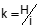## 1. Field Conditions

Select an open area that allows a flat horizontal sight for up to 150 feet. Shorter distances are better to minimize rod reading errors.

A windless day is preferred.

The telescope of a  transit or theodolite should be locked in a horizontal orientation. Using an inclined LoS can result in unequal half-intervals, Figure D-1.

Use a level rod with with either an attached bubble or separate hand held bubble - be sure the bubble is in adjustment.

Though not required, a rod bipod provides a steady rod setup.(a) Inclined: t>b (b) Depressed: t

## 2. Checks

The checks in this chapter are for internal focusing instruments. The objective lens of an internal focus instrument is fixed; focusing is accomplished using internal lenses. The objective lens of an external focus instrument moves as the telescope is focused changing its optical geometry. External focus instruments require a constant (generally equal to 1) be added to stadia slope distance. Because external focus instruments are obsolete, they aren't covered in this chapter.

### a. Vertical Circle Index

#### (1) Description

Reducing slope distance to horizontal requires measurement of a vertical or zenith angle. An index error exists if the vertical circle or Beaman arc is not correctly oriented to gravity when the instrument is leveled.

This check and adjustment is described in Chapter G Theodolite and Total Stations.

#### (1) Description

The stadia multiplier is used determine the horizontal distance to a level rod. Modern instruments do not require a stadia constant and generally have a multiplier of 100 (with some exceptions).

#### (2) Check

The check uses known distances to compute the multiplier. Level rod readings at longer distances are subject to larger random errors. For multiplier determination, shorter distances should be used to minimize reading errors. Although only a single known distance is needed, more of varying lengths allow for a better multiplier determination.

Distances should be known to an accuracy higher than stadia's. Stadia absolute accuracy is limited to about 1 foot (0.01 foot rod division) or 10 cm (0.001 meter rod division). Distances up to about 200 feet can be laid out with a fiberglass tape with sufficient accuracy if carefully done.

The procedure described here uses two distances observed twice, Figure D-2.Figure D-2Using Two Distances

The multiplier is determined using Equation D-1.Equation D-1

Interpolate readings to half-interval if possible to do so consistently. This will help minimize reading errors.

(a) Set three points, A, B, and C.

Point A will be the instrument location.

Point B will be a rod location and should be 50 to 75 feet from point A

Point C will be a rod location and should be 75 to 150 feet from point A and at least 25 feet from point B.

The points do not need to be in a straight line.

(b) Using a tape, EDM, or TSI, measure the horizontal distances from point A to point B and point C.

(c) Set up the instrument over point A.

Read and record the three wires on the rod at point B.

Read and record the three wires on the rod at point C.

(d) Take down the instrument and re-set it over point A (for a second independent measurement set)

Read and record the three wires on the rod at point B.

Read and record the three wires on the rod at point C.

(e) Use Equation D-6 to compute the multiplier for the four observations distances

Compute the average multiplier and compare to the manufacturer's specified value. The multiplier should be computed to the nearest whole number.

Figure D-3 is a sample note form for two distances and two setups.Figure D-3Sample Note Form

Hits: 565